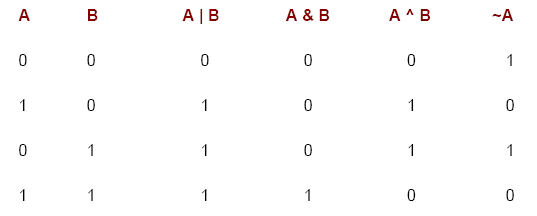# Programming Tutorials

## Bitwise logical operators in Java

The bitwise logical operators are &, |, ^, and ~. The following table shows the outcome of each operation. In the discussion that follows, keep in mind that the bitwise operators are applied to each individual bit within each operand.

The Bitwise NOT

Also called the bitwise complement, the unary NOT operator, ~, inverts all of the bits of its operand. For example, the number 42, which has the following bit pattern:

```00101010 becomes 11010101```

after the NOT operator is applied.

The Bitwise AND

The AND operator, &, produces a 1 bit if both operands are also 1. A zero is produced in all other cases. Here is an example:

00101010 42
&00001111 15
----------
00001010 10

The Bitwise OR

The OR operator, |, combines bits such that if either of the bits in the operands is a 1, then the resultant bit is a 1, as shown here:

```00101010 42 | 00001111 15 v 00101111 47```

The Bitwise XOR

The XOR operator, ^, combines bits such that if exactly one operand is 1, then the result is 1. Otherwise, the result is zero. The following example shows the effect of the ^. This example also demonstrates a useful attribute of the XOR operation. Notice how the bit pattern of 42 is inverted wherever the second operand has a 1 bit. Wherever the second operand has a 0 bit, the first operand is unchanged. You will find this property useful when performing some types of bit manipulations.

```00101010 42 ^00001111 15 ---------- 00100101 37```Using the Bitwise Logical Operators

The following program demonstrates the bitwise logical operators:

```// Demonstrate the bitwise logical operators. class BitLogic { public static void main(String args[]) { String binary[] = { "0000", "0001", "0010", "0011", "0100", "0101", "0110", "0111", "1000", "1001", "1010", "1011", "1100", "1101", "1110", "1111" }; int a = 3; // 0 + 2 + 1 or 0011 in binary int b = 6; // 4 + 2 + 0 or 0110 in binary int c = a | b; int d = a & b; int e = a ^ b; int f = (~a & b) | (a & ~b); int g = ~a & 0x0f; System.out.println(" a = " + binary[a]); System.out.println(" b = " + binary[b]); System.out.println(" a|b = " + binary[c]); System.out.println(" a&b = " + binary[d]); System.out.println(" a^b = " + binary[e]); System.out.println("~a&b|a&~b = " + binary[f]); System.out.println(" ~a = " + binary[g]); } }```

In this example, a and b have bit patterns which present all four possibilities for two binary digits: 0-0, 0-1, 1-0, and 1-1. You can see how the | and & operate on each bit by the results in c and d. The values assigned to e and f are the same and illustrate how the ^ works. The string array named binary holds the human-readable, binary representation of the numbers 0 through 15. In this example, the array is indexed to show the binary representation of each result. The array is constructed such that the correct string representation of a binary value n is stored in binary[n]. The value of ~a is ANDed with 0x0f (0000 1111 in binary) in order to reduce its value to less than 16, so it can be printed by use of the binary array. Here is the output from this program:

```a = 0011 b = 0110 a|b = 0111 a&b = 0010 a^b = 0101 ~a&b|a&~b = 0101 ~a = 1100```